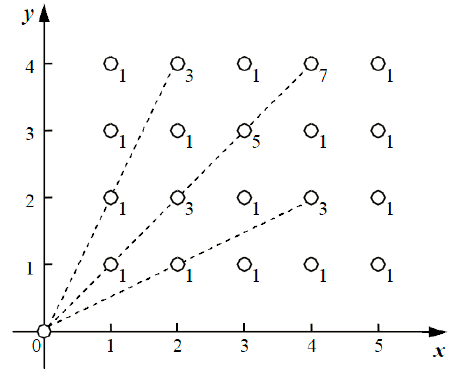## Day 1, Problem 1 - Energy Harvesting

DongDong owns a rectangular strip of land. On it, he can plant a special type of energy plant with ability to harvest energy from the sun. After the plants have harvested the energy, DongDong will use an energy pooling machine to gather all the solar energy collected by the plants to one place.

DongDong's plants are neatly arranged arranged into n rows, with m plants in each row. The vertical and horizontal distances between adjacent plants are all the same. Thus, each of DongDong's plants can be represented with the coordinates (x, y), where x can be from 1 to n, and y can be from 1 to m, indicating that the plant is in column y of row x.

Since the energy pooling machine is rather large and hard to move, DongDong has placed it into a corner with the coordinates (0, 0). In the process of pooling energy, a certain amount of energy is bound to be lost. If the line segment formed between a plant and the pooling machine intersects with k other plants, then the energy lost will be 2k + 1 units. For example, the machine is collecting energy from the plant at (2, 4), but since one plant at (2, 1) is on the line segment between them, the energy lost will be 3. Note: if there are no other plants on the line segment, then 1 unit of energy will be lost. Now, you must determine the total energy loss of the pooling process.

Following is an example of energy pooling for n = 5 and m = 4. There are a total of 20 plants. The number labeled beside each plant represents the energy loss for that plant.In this example, the total energy lost is 36.

### Input Format

The input consists of a single line with two integers n and m.

### Output Format

The output should consist of a single integer, representing the total energy loss.

`5 4`

`36`

`3 4`

`20`

### Constraints

For 10% of test cases: 1 ≤ n, m ≤ 10.
For 50% of test cases: 1 ≤ n, m ≤ 100.
For 80% of test cases: 1 ≤ n, m ≤ 1000.
For 90% of test cases: 1 ≤ n, m ≤ 10,000.
For 100% of test cases: 1 ≤ n, m ≤ 100,000.

Point Value: 17 (partial)
Time Limit: 1.00s
Memory Limit: 512M